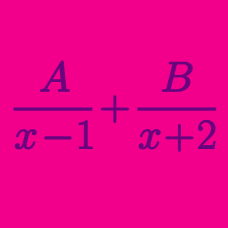Algebra

Partial Fractions - Linear Factors

If the following is an identity in $x$: $\frac{A}{(x-2)(5x-8)}=\frac{1}{x-2}-\frac{B}{5x-8},$ what is the value of $A+B?$

How many terms would there be in the partial fraction decomposition of

$\frac{1}{ ( x- 5) ( x + 5) ( x + 9 )}?$

If the following is an identity in $x$:

$\frac{11x+50 }{(x+2)(x+6)} = \frac{A}{x+2} + \frac{B}{x+6},$

what is the value of $A + B?$

Which of the following is the correct partial fraction decomposition of

$\frac{ x+1 } { ( x + 2) ( x + 3) } ?$

If the following is an identity in $x$: $\frac{5x-6}{x^2-3x+2}=\frac{a}{x-1}+\frac{b}{x-2},$ what is the value of $a \times b$?

×